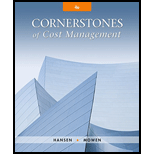# The following if-then statements were taken from a Balanced Scorecard: a. If employee capabilities increase, then process time decreases. b. If process time decreases, then customer retention will increase. c. If customer retention increases, then market share will increase. d. If market share increases, then revenues will increase. Required: 1. Identify the lead and lag variables, and explain your reasoning. 2. Discuss the implications of Requirement 1 for the financial and learning and growth perspectives. 3. Using the first if-then statement, explain the concept of double-loop feedback.### Cornerstones of Cost Management (C...

4th Edition
Don R. Hansen + 1 other
Publisher: Cengage Learning
ISBN: 9781305970663

#### Solutions

Chapter
Section### Cornerstones of Cost Management (C...

4th Edition
Don R. Hansen + 1 other
Publisher: Cengage Learning
ISBN: 9781305970663
Chapter 13, Problem 11E
Textbook Problem
10 views

## The following if-then statements were taken from a Balanced Scorecard: a. If employee capabilities increase, then process time decreases. b. If process time decreases, then customer retention will increase. c. If customer retention increases, then market share will increase. d. If market share increases, then revenues will increase. Required: 1. Identify the lead and lag variables, and explain your reasoning. 2. Discuss the implications of Requirement 1 for the financial and learning and growth perspectives. 3. Using the first if-then statement, explain the concept of double-loop feedback.

1.

To determine

Identify the lead and lag variables.

### Explanation of Solution

Lead indicators: Lead indicators of measures of nonfinancial and financial outcomes that direct management for taking current decisions that result in enviable results in the future.

Lag indicators: Lag indicators of final outcomes of previous decisions of management.

A lead indicator enables outcome measures that are to be attained. Certain measures acts as both “lead and lag indicators”. Without expressing the underlying strategy as a series of “if-then statements”, then it becomes very complex to categorize measures as lead or lag. For example, if A, then B, if B, then C, helps to recognize the “lead and lag roles of measures”. If a measure is related with a premise, then it works as a lag variable and if a measure is related to a consequence, it functions as a lab measure...

2.

To determine

Discuss the implications of requirement 1 for the financial and learning and growth perspectives.

3.

To determine

Explain the concept of “double-loop feedback”, using the first “if-then statement”.

### Still sussing out bartleby?

Check out a sample textbook solution.

See a sample solution

#### The Solution to Your Study Problems

Bartleby provides explanations to thousands of textbook problems written by our experts, many with advanced degrees!

Get Started